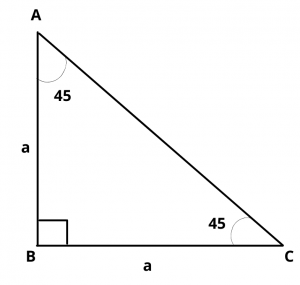# What is the Value of Cosec 45 Degrees ?

## Solution :

The value of cosec 45 degrees is $$\sqrt{2}$$.

Proof :

Let ABC be a triangle, right angled at B, in which $$\angle$$ A = $$\angle$$ C = 45 degrees$$\therefore$$  BC = AB

Let  AB = BC = a

Then by pythagoras theorem,

$$AC^2$$ = $$AB^2$$ + $$BC^2$$ = $$a^2$$ + $$a^2$$ = $$2a^2$$

$$\implies$$  AC = $$\sqrt{2}a$$

In $$\Delta$$ ABC,  $$\angle$$ C = 45 degrees

By using trigonometric formulas,

$$cosec 45^{\circ}$$ = $$hypotenuse\over perpendicular$$ = $$h\over p$$

$$cosec 45^{\circ}$$  = hypotenuse/side opposite to 45 degrees = $$AC\over AB$$ = $$\sqrt{2}a\over a$$ = $$\sqrt{2}$$

Hence, the value of $$cosec 45^{\circ}$$ = $$\sqrt{2}$$